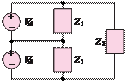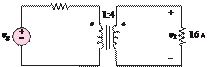### Create an Account

Already have account?

### Forgot Your Password ?

Home / Questions / For the circuit shown in Figure P743 assume that vg 120 V rms Find a The total resi...

# For the circuit shown in Figure P743 assume that vg 120 V rms Find a The total resistance seen by the voltage source b The primary current c The primary power

For the circuit shown in Figure P7.43, assume that

vg  = 120 V rms. Find

a.    The total resistance seen by the voltage source.

b.    The primary current.

c.    The primary power.Figure   P7.39                                                                                     1 Ù

View less »

Jun 18 2020 View more View Less

#### Answer (Solved)Subscribe To Get Solution